## Plus Blog

December 14, 2013

A polyhedron is the 3D version of a polygon. It's a solid object whose surface is made up of a number of polygonal faces. Two faces meet in an edge and the corners of a polyhedron are called vertices.

Euler's polyhedron formula, named after Leonhard Euler, is a pretty amazing equation relating the number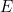of edges,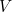of vertices and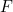of faces of a polyhedron: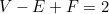As an example, think of a cube. It's got 6 faces, 8 vertices and 12 edges: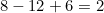as required.

The amazing thing is that this formula holds for all polyhedra, except for those that have holes running through them.

Using this formula, you can figure out quickly that there is no simple polyhedron (that is one without holes) with exactly seven edges. Similarly, there is no simple polyhedron with ten faces and seventeen vertices.

The formula also makes it possible to prove one of the most beautiful results in geometry, that there are only five Platonic solids.Figure 7: The Platonic solids. From left to right we have the tetrahedon with four faces, the cube with six faces, the octahedron with eight faces, the dodecahedron with twelve faces, and the icosahedron with twenty faces.

Euler's polyhedron formula applies to solids that, in a topological sense, are equivalent to the sphere: you can turn each simple polyhedron into a sphere by smoothing out the edges and corners and making it round. But you can also look at the number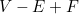for polyhedra that are topologically the same as a doughnut, or a doughnut with two, three, four or any number of holes. This number is called the Euler characteristic and it turns out that it is always equal to 2 minus 2 times the number of holes. So the Euler characteristic doesn't only tell us something about polyhedra, but also something very deep about the nature of shape and space in general.

You can find out more about Euler's polyhedron formula, including a proof, in this Plus article. And there's an interesting application to designing footballs in A fly walks around a football.

December 13, 2013

No, this isn't about Homer, but it's interesting anyway. Suppose you're trying to decide which university to go to. You find out that last year the university you're interested in admitted 30% of male applicants but only 21.3% of female applicants. Looks like a clear case of gender bias, so you're tempted to go somewhere else. But then you look at the figures again, this time broken up by department. The university only has two departments, maths and English. The English department admitted 40% of male applicants and 42% of female applicants. The maths department admitted 10% of male applicants and 11% of female applicants. So if you look at the figures by department, if anything there's bias in favour of women. What's going on?This is an example of Simpson's paradox, which arises when you look at percentages without giving the actual numbers involved. Suppose the English department admits quite a high proportion of applicants, while the maths department is more choosy and admits only a small proportion. Now suppose that most of the male applicants apply to the English department. Then this drives up the overall percentage of successful male applicants, as English is easier to get into. Similarly, if most women apply to the maths department, then this lowers the overall percentage of successful women applicants, because maths is harder to get into. So it can happen that, although both departments favour women, the overall percentage of successful female applicants is lower than that for males.

Let's go back to the example: suppose that 100 men apply to the English department, so that means that 40 of them got in (40%). Suppose that only 50 women applied to the English department, so 21 of them got in (42%). Suppose the maths department had only 50 male applicants, so 5 got in (10%), and 100 female applicants, of which 11 got in (11%). Then the overall proportion of male applicants who were successful is 45/150 corresponding to 30%. For the women the overall proportion is 32/150 corresponding to 21.3%. Mystery solved.

This paradox isn't just a theoretical curiosity. In 1973 The University of California at Berkeley was sued for sex bias on the basis of figures that were an illustration of Simpson's paradox. It turned out that on the whole women had applied to more competitive departments and that's how the seemingly biased figures arose.

If it's the other Simpsons you're interested in have a look at Simon Singh's book.

December 12, 2013

There's nothing simpler than 1,2,3, ... we understand these numbers instinctively and that's why they're called the natural numbers. But if you really think about it, what are these numbers? How would you describe them to an alien devoid of a number instinct? Here's one way of defining them, developed by the Italian mathematician Giuseppe Peano: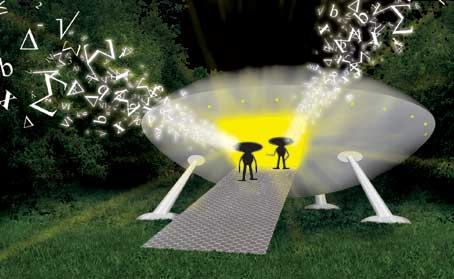Explaining numbers to aliens.

1. First you proclaim that 1 is a natural number
2. Then you say that every natural number n has a successor s(n), which you can also write as n+1.
3. We also insist that this successor is never equal to 1
4. And that different numbers have different successors.

These four rules give you all the natural numbers, neatly ordered in a line, starting from 1 (you could also have started from 0). They also give you arithmetic, since addition and multiplication are about repeatedly adding 1s and you know how to do this: you simply move up to the successor of the number you're looking at. Subtraction and division are just the reverse of addition and multiplication. So equipped with these rules your innumerate alien could actually do some pretty decent number theory.

The four rules form the basis of what's called Peano arithmetic. It's a formal mathematical system based on a set of axioms (which includes these four rules) together with a language in which to speak about numbers and rules for logical inference. In the beginning of the 20th century mathematicians hoped they could turn all of maths into one giant formal system similar to Peano's arithmetic. That way they could prove everything directly from the axioms, without any hidden assumptions, and make sure that maths contains no contradictions. But their dream was shattered in the 1930s by the logician Kurt Gödel, who showed that there are logical limits to what you can do using formal systems. Find out more in

December 10, 2013If it wasn't for the Babylonians who lived millennia ago, paying your bill at a restaurant or checking your credit card bill would be much more painful than it already is. And so would anything else involving numbers. It was these Babylonian scholars who had the brilliant idea of making the value of a numeral dependent on its position in a string of numerals: if you see a 1 at the end of a string, you know it means 1, if it's shifted one to the left, you know it means 10, if it's shifted two to the left, it means 100 and so on. This means that using only the symbols 0 to 9 you can write down every single whole number, no matter how large, rather than having to invent a new symbol every time you go up a order of magnitude.

The Babylonian number system actually had 60 as its base, rather than base ten as we do. The numerals we use today, including the use of a symbol (zero) for nothing instead of a gap, stems from India.

Find out more on in the Fabulous positional system and out other articles about number systems.

December 9, 2013

I went Christmas shopping yesterday, to buy a book from the local bookshop that costs £7. I didn't have any money, so I borrowed £5 from Marianne and £5 from Owen. I bought the book and got £3 change. I gave £1 back to each of my kind colleagues and I keep the remaining £1. I now owe each of them £4 and I have £1, giving £9 in total. But I borrowed £10. Where's the missing pound?There it is!

The answer is that the £10 are a red herring. There's no reason why the money I owe after the whole transaction and the money I still have should add up to £10. Rather, the money I owe minus the change I got should come to the price of the book, that is £7. Giving a pound back to each of Marianne and Owen just re-distributes the amounts. The money I still owe is reduced to £8 and the money I still have to £1. Rather than having £10-£3=£7, we now have £8-£1=£7. Mystery solved!

December 8, 2013

An infinite set is called countable if you can count it. In other words, it's called countable if you can put its members into one-to-one correspondence with the natural numbers 1, 2, 3, ... . For example, a bag with infinitely many apples would be a countable infinity because (given an infinite amount of time) you can label the apples 1, 2, 3, ... and so on.

Two countably infinite sets A and B are considered to have the same "size" (or cardinality) because you can pair each element in A with one and only one element in B so that no elements in either set are left over. This idea seems to make sense, but it has some funny consequences. For example, the even numbers are a countable infinity because you can link the number 2 to the number 1, the number 4 to 2, the number 6 to 3 and so on. So if you consider the totality of even numbers (not just a finite collection) then there are just as many of them as natural numbers, even though intuitively you'd think there should only be half as many.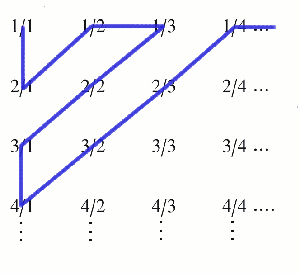Something similar goes for the rational numbers (all the numbers you can write as fractions). You can list them as follows: first write down all the fractions whose denominator and numerator add up to 2, then list all the ones where the sum comes to 3, then 4, etc. This is an unfailing recipe to list all the rationals, and once they are listed you can label them by the natural numbers 1, 2, 3, ... . So there are just as many rationals as natural numbers, which again seems a bit odd because you'd think that there should be a lot more of them.

It was Galileo who first noticed these funny results and they put him off thinking about infinity. Later on the mathematician Georg Cantor revisited the idea. In fact, Cantor came up with a whole hierarchy of infinities, one "bigger" than the other, of which the countable infinity is the smallest. His ideas were controversial at first, but have now become an accepted part of pure mathematics.

You can find out more about all this in our collection of articles on infinity.